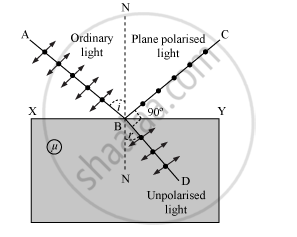# Show, via a Suitable Diagram, How Unpolarised Light Can Be Polarised by Reflection. - Physics

Show, via a suitable diagram, how unpolarised light can be polarised by reflection.

#### SolutionAn ordinary beam of light on reflection from a transparent medium becomes partially polarised. The degree of polarisation increases as the angle of incidence is increased. At a particular value of angle of incidence, the reflected beam becomes completely polarised. This angle of incidence is called the polarising angle (p).

When light is incident at polarising angle at the interface of a refracting medium, the refractive index of the medium is equal to the tangent of the polarising angle.

μ = tan p

where

μ → Refractive index of the refracting medium

p → Polarising angle

Concept: Polarisation
Is there an error in this question or solution?